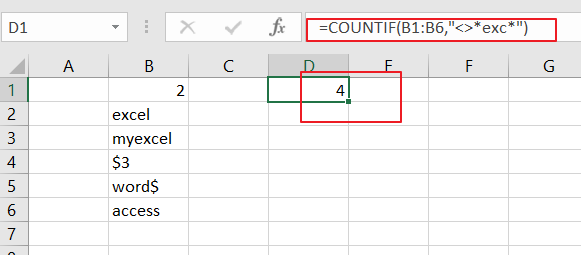How to Count Cells that do not Contain Specific Text in Excel

In the previous post, we talked that how to count cell that contain certain text using the COUNTIF function in Excel.

This post will guide you how to count the number of cells that do not contain specific text within a range of cells using a formula in Excel 2013/2016 or Excel office 365. How do I count the number of cells without certain text using a simple formula in Excel. When you analyze a large list of data, you may also want to know that how many cells that do not contain specific text. The below steps will show you how to do it in a simple way.

Count Number of Cells that do Not Contain Specific Text

You can use the COUNTIF function to count cells that do not contain certain text. And you also need to supply the target text string in the criteria argument in the COUNTIF function.

The below is a generic formula to count the number of cells that do not contain specific text:

=COUNTIF(range,criteria)

Assuming that you have a list of data in range B1:B6 and you want to count the number of cells without a certain text “exc”. And you can type this string in the second argument as its criteria and you will get the below formula:

=COUNTIF(B1:B6,”<>*exc*”)LET’S SEE THAT HOW THIS FORMULA WORKS:

The COUNTIF function can be used to count the number of cells that match a single condition or criteria. And in this case, you need to provide a criteria as “<>*exc*”, which is evaluated as “values that do not contain ‘exc’ in any position”. Then the total count of all cells in the range B1:B6 that meet the above criteria is returned.

Note: the asterisk(*) means that it will match any number of characters in the above formula. If you just only supply a certain string “exc”, it means that cells must contain exactly “exc”. If the cell contains any other characters, it will not be counted. So you need to use the asterisk character as a wildcard in the second criteria argument.

The “<>” operator means that does not equal to a certain value, so the above formula can be used to count any cell that does not contain “exc” in any position in cells.

Related Functions

• Excel COUNTIF function
The Excel COUNTIF function will count the number of cells in a range that meet a given criteria. This function can be used to count the different kinds of cells with number, date, text values, blank, non-blanks, or containing specific characters. etc. = COUNTIF (range, criteria) …

Related Posts

Filter And Transpose Data From Horizontal To Vertical

This post will show you how to use Filter function and in combination with Transpose function to filter data from horizontal and transpose data as vertical in Microsoft Excel. You can refer to the below general formula based on TRANSPOSE ...

VLOOKUP Formula | Faster Trick with 2 VLOOKUPS

This post will guide you how to use 2 VLOOKUPS function to looking up data entries from a given range of cells in Microsoft Excel. VLOOKUP with 2 lookups can be faster than a single VLOOKUP in certain scenarios. The ...

Extract Unique Items From A List

This post will guide you how to extract unique itmes from a given list in Microsoft Excel. How to create a newly formula to get unique values from a range cells in Excel. The unique list of items is the ...

Extract substring In Excel

This post will guide you how to use Excel's MID function is a quick and easy way to extract pieces from your text. Use the Excel formula to extract a substring with MID. Note: If you want to extract just ...

How to Use 3D SUM Multiple Worksheets

To sum a range of numbers is straightforward for most Excel users, but do you know how to establish a 3D reference to total the same range of numerous sheets. In this post, I will present the steps for this ...

Extract all the matches with helper Column

With Excel's powerful functions IF, INDEX, and MATCH, we can find exactly what you're looking for with a few clicks of the mouse. This step-by-step tutorial will show how easy it is to extract data using these tools and more! ...

If Cell is This Value or That Value

IF function is frequently used in Excel worksheet to return you expect “true value” or “false value” based on the result of logical test. If you want to see if a cell is A or B, and if one of ...

If Value is Greater Than A Certain Value

IF function is frequently used in Excel worksheet to return you expect “true value” or “false value” based on the logical test result. If you want to see if a value in one cell is greater than a specific value, ...

If Cell is Not Blank

IF function is frequently used in Excel worksheet to return you expect “true value” or “false value” based on the result of created logical test. If you want to see if a cell is blank or not, and leave some ...

If Cell is Blank

IF function is frequently used in Excel worksheet to return you expect “true value” or “false value” based on the result of created logical test. If you want to see if a cell is blank or not, and leave some ...

Sidebar